1. /
2. CBSE
3. /
4. Class 07
5. /
6. Mathematics
7. /
8. NCERT Solutions for Class...

# NCERT Solutions for Class 7 Maths Exercise 10.2### myCBSEguide App

Download the app to get CBSE Sample Papers 2023-24, NCERT Solutions (Revised), Most Important Questions, Previous Year Question Bank, Mock Tests, and Detailed Notes.

NCERT solutions for Maths Practical Geometry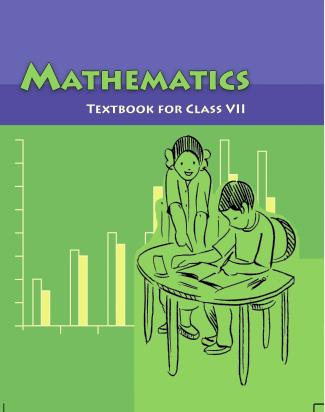## NCERT Solutions for Class 7 Maths Practical Geometry

Class –VII Mathematics (Ex. 10.2)

###### Question 1.Construct {tex}\Delta {/tex}XYZ in which XY = 4.5 cm, YZ = 5 cm and ZX = 6 cm.

To construct: {tex}\Delta {/tex}XYZ, where XY = 4.5 cm, YZ = 5 cm and ZX = 6 cm.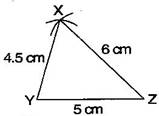Steps of construction:

(a) Draw a line segment YZ = 5 cm.

(b) Taking Z as centre and radius 6 cm, draw an arc.

(c) Similarly, taking Y as centre and radius 4.5 cm, draw another arc which intersects first arc at point X.

(d) Join XY and XZ.

It is the required {tex}\Delta {/tex}XYZ.

###### Question 2.Construct an equilateral triangle of side 5.5 cm.

To construct: A {tex}\Delta {/tex}ABC where AB = BC = CA = 5.5 cm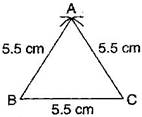Steps of construction:

(a) Draw a line segment BC = 5.5 cm

(b) Taking points B and C as centers and radius 5.5 cm, draw arcs which intersect at point A.

(c) Join AB and AC.

It is the required {tex}\Delta {/tex}ABC.

NCERT Solutions for Class 7 Maths Exercise 10.2

###### Question 3.Draw {tex}\Delta {/tex}PQR with PQ = 4 cm, QR = 3.5 cm and PR = 4 cm. What type of triangle is this?

To construction: {tex}\Delta {/tex}PQR, in which PQ = 4 cm, QR = 3.5 cm and PR = 4 cm.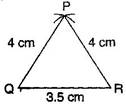Steps of construction:

(a) Draw a line segment QR = 3.5 cm.

(b) Taking Q as centre and radius 4 cm, draw an arc.

(c) Similarly, taking R as centre and radius 4 cm, draw an another arc which intersects first arc at P.

(d) Join PQ and PR.

It is the required isosceles {tex}\Delta {/tex}PQR.

NCERT Solutions for Class 7 Maths Exercise 10.2

###### Question 4.Construct {tex}\Delta {/tex}ABC such that AB = 2.5 cm, BC = 6 cm and AC = 6.5 cm. Measure {tex}\angle {/tex}B.

To construct :{tex}\Delta {/tex}ABC in which AB = 2.5 cm, BC = 6 cm and AC = 6.5 cm.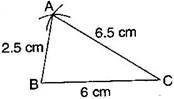Steps of construction:

(a) Draw a line segment BC = 6 cm.

(b) Taking B as centre and radius 2.5 cm, draw an arc.

(c) Similarly, taking C as centre and radius 6.5 cm, draw another arc which intersects first arc at point A.

(d) Join AB and AC.

(e) Measure angle B with the help of protractor.

It is the required {tex}\Delta {/tex}ABC where {tex}\angle {/tex}B = {tex}80^\circ .{/tex}

## NCERT Solutions for Class 7 Maths Exercise 10.2

NCERT Solutions Class 7 Maths PDF (Download) Free from myCBSEguide app and myCBSEguide website. Ncert solution class 7 Maths includes text book solutions from Class 7 Maths Book . NCERT Solutions for CBSE Class 7 Maths have total 15 chapters. 7 Maths NCERT Solutions in PDF for free Download on our website. Ncert Maths class 7 solutions PDF and Maths ncert class 7 PDF solutions with latest modifications and as per the latest CBSE syllabus are only available in myCBSEguide.

## CBSE app for Students

To download NCERT Solutions for Class 7 Maths, Social Science Computer Science, Home Science, Hindi English, Maths Science do check myCBSEguide app or website. myCBSEguide provides sample papers with solution, test papers for chapter-wise practice, NCERT solutions, NCERT Exemplar solutions, quick revision notes for ready reference, CBSE guess papers and CBSE important question papers. Sample Paper all are made available through the best app for CBSE students and myCBSEguide website.Test Generator

Create question paper PDF and online tests with your own name & logo in minutes.myCBSEguide

Question Bank, Mock Tests, Exam Papers, NCERT Solutions, Sample Papers, Notes

### 3 thoughts on “NCERT Solutions for Class 7 Maths Exercise 10.2”

1. very good and useful

2. It’s very helpful for students like me

3. Help full app